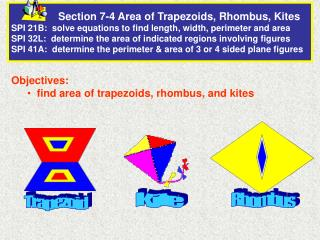DownloadDownload PresentationObjectives: find area of trapezoids, rhombus, and kites

Objectives: find area of trapezoids, rhombus, and kites

Download PresentationObjectives: find area of trapezoids, rhombus, and kites

- - - - - - - - - - - - - - - - - - - - - - - - - - - E N D - - - - - - - - - - - - - - - - - - - - - - - - - - -
Presentation Transcript

1. Section 7-4 Area of Trapezoids, Rhombus, Kites SPI 21B: solve equations to find length, width, perimeter and areaSPI 32L: determine the area of indicated regions involving figuresSPI 41A: determine the perimeter & area of 3 or 4 sided plane figures • Objectives: • find area of trapezoids, rhombus, and kites Kite Rhombus Trapezoid

2. Area and Perimeter of a Trapezoid Trapezoid A = ½ h(b1 + b2) • Height: • the perpendicular distance h between the bases. • To find Perimeter: • add the lengths of the sides • may need to use • Pythagorean Thm • 30-60-90 Triangle Thm • 45-45-90 Triangle Thm

3. 1 2 A = h(b1 + b2) Area of a trapezoid 1 2 A = (18)(20 + 36) Substitute 18 for h, 20 for b1, and 36 for b2. Find the Area of a Trapezoid A car window is shaped like the trapezoid shown. Find the area of the window. A = 504 Simplify. The area of the car window is 504 in.2

4. Find the Area of a Trapezoid Find the area of trapezoid ABCD. Draw an altitude from vertex B to DC that divides trapezoid ABCD into a rectangle and a right triangle. Because opposite sides of rectangle ABXD are congruent, DX = 11 ft XC = 16 ft – 11 ft = 5 ft.

5. 1 2 A = h(b1 + b2) Use the trapezoid area formula. 1 2 A = (12)(11 + 16) Substitute 12 for h, 11 for b1, and 16 for b2. (continued) By the Pythagorean Theorem, BX2 + XC2 = BC2, so BX2 = 132 – 52 = 144. Taking the square root, BX = 12 ft. A = 162 Simplify. The area of trapezoid ABCD is 162 ft2.

6. Area and Perimeter of a Rhombus or Kite Rhombus or Kite Area = ½ d1d2 d is the diagonal • To find Perimeter: • add the lengths of the sides

7. Find the Area of a Kite Find the area of kite XYZW. Kite: 2 pairs of adjacent congruent sides. Opposite sides not congruent Find the lengths of the diagonals of kite XYZW. XZ = d1 = 3 + 3 = 6 and YW = d2 = 1 + 4 = 5 1 2 A = d1d2Use the formula for the area of a kite. 1 2 A = (6)(5) Substitute 6 for d1 and 5 for d2. A = 15 Simplify. The area of kite XYZW is 15 cm2.

8. Find the Area of a Kite Find the area of rhombus RSTU. To find the area, you need to know the lengths of both diagonals. Draw diagonal SU, and label the intersection of the diagonals point X.

9. SXT is a right triangle because the diagonals of a rhombus are perpendicular. 1 2 A = d1d2Area of a rhombus 1 2 A = (24)(10) Substitute 24 for d1 and 10 for d2. … continued The diagonals of a rhombus bisect each other, so TX = 12 ft. You can use the Pythagorean triple 5, 12, 13 or the Pythagorean Theorem to conclude that SX = 5 ft. SU = 10 ft because the diagonals of a rhombus bisect each other. A = 120 Simplify. The area of rhombus RSTU is 120 ft2.